# R S Aggarwal Solutions for Class 11 Maths Chapter 21 Circles Exercise 21B

R S Aggarwal Solutions Class 11 Maths are given here for Chapter 21 – Circles exercise 21B. We are providing R S Aggarwal Chapter 21 solutions to help students go through and practice several solved problems. In this exercise, students will study about general equation of a circle.

Important Note:

The general equation of a circle is of the form x2 + y2 + 2gx + 2fy + c = 0.

Where (-g, -f) is the centre and radius = sqrt(g2 + f2 – c)

Also, every equation of the form x2 + y2 + 2gx + 2fy + c = 0 represents a circle if (g2 + f2 – c) > 0.

Find detailed solutions for chapter 21 exercise 21B below.

## Download PDF of R S Aggarwal Solutions for Class 11 Maths Chapter 21 Circles Exercise 21B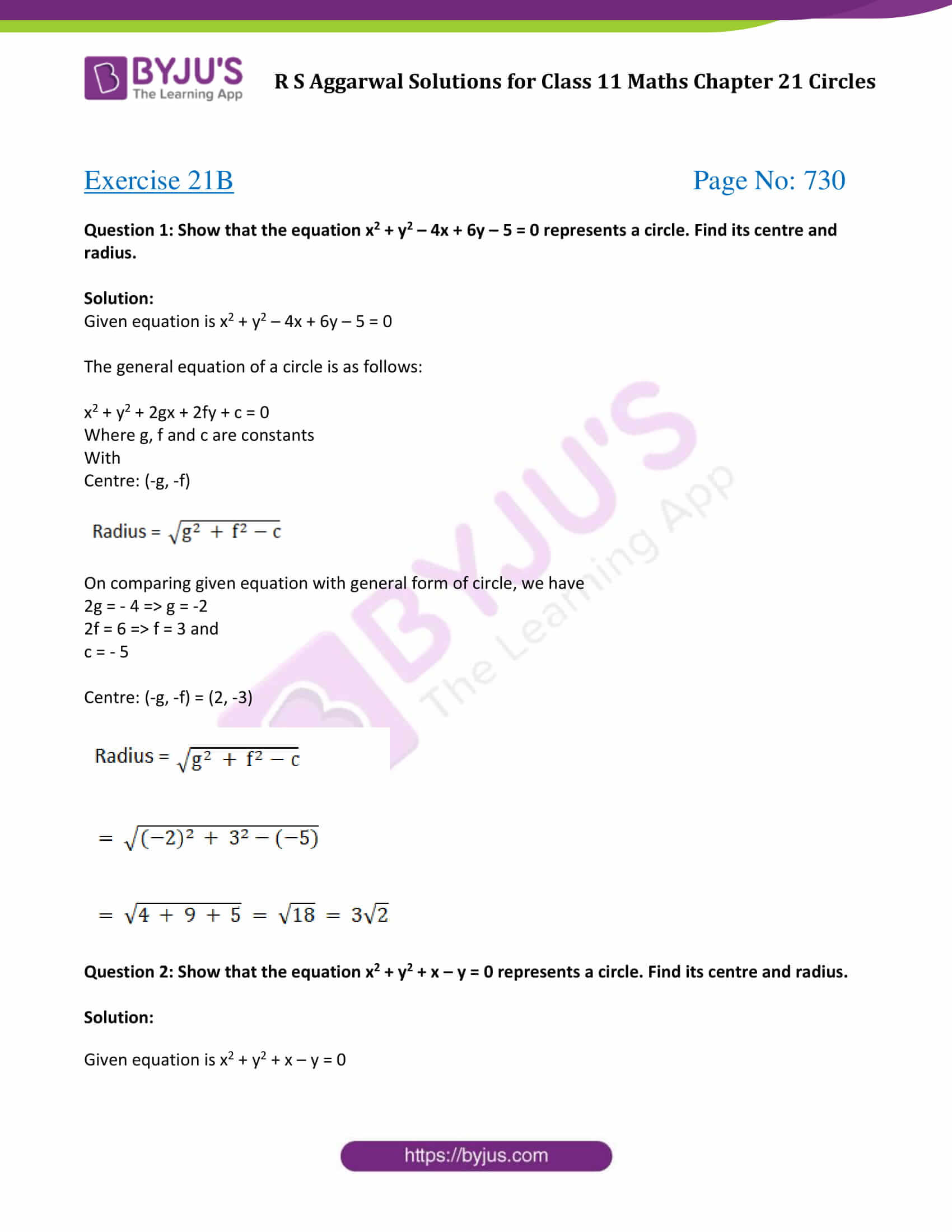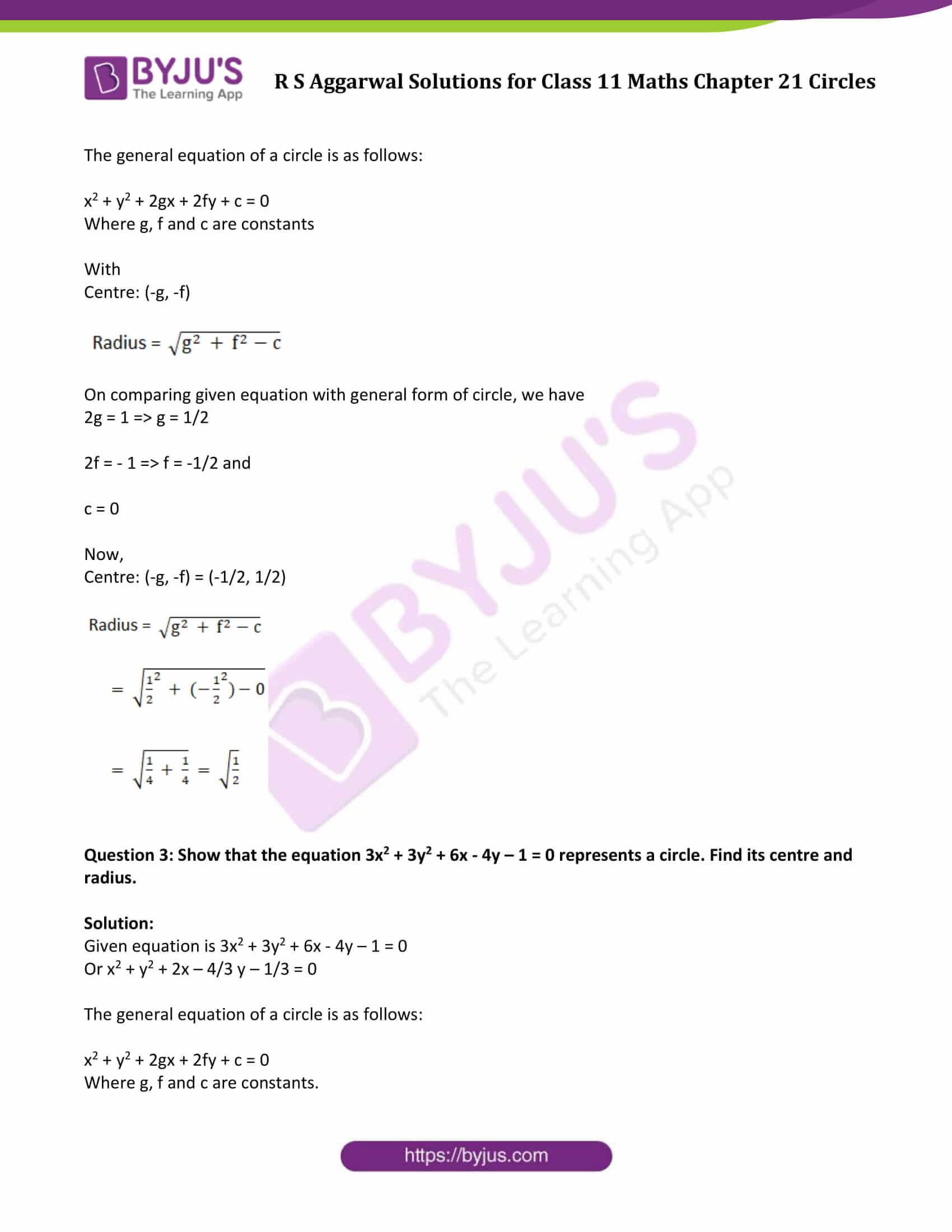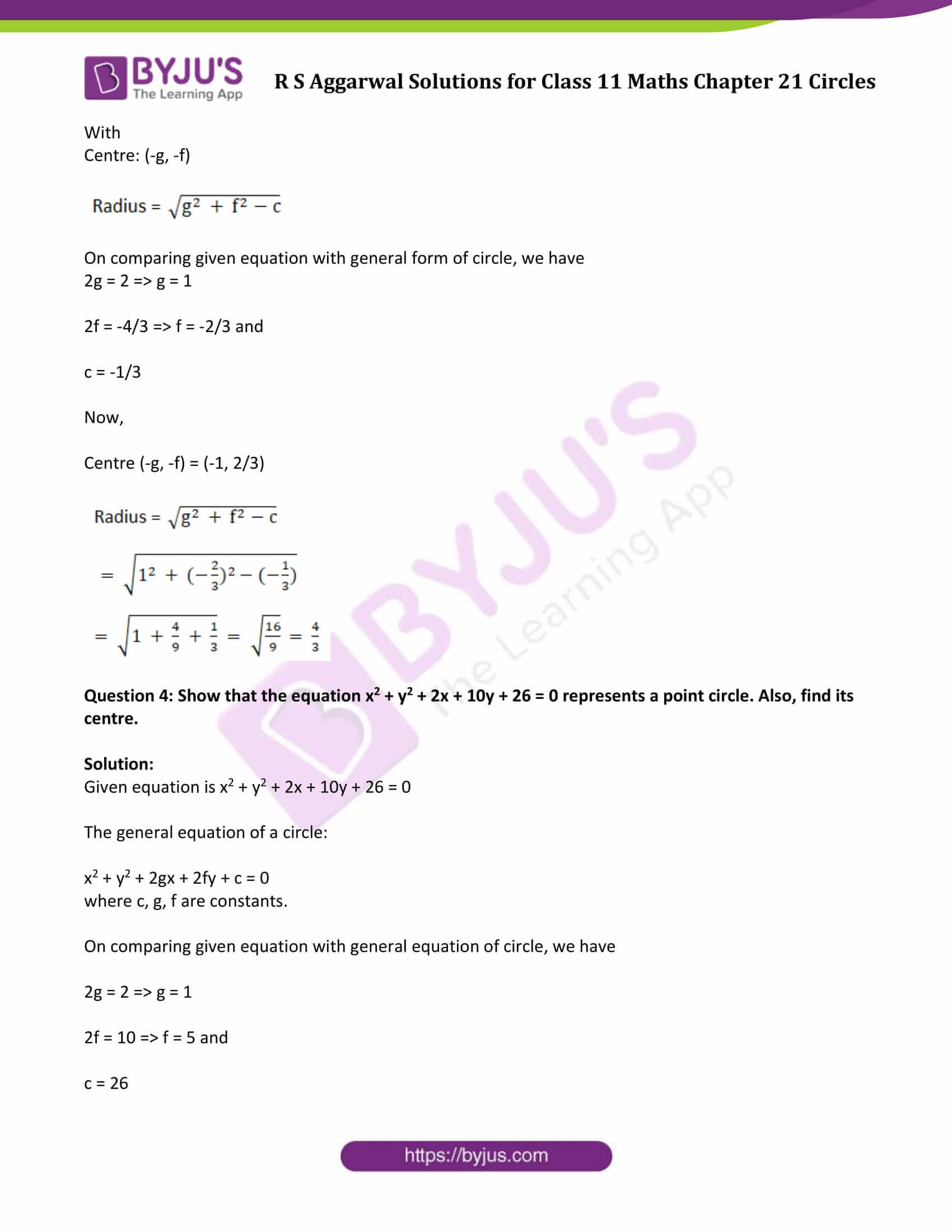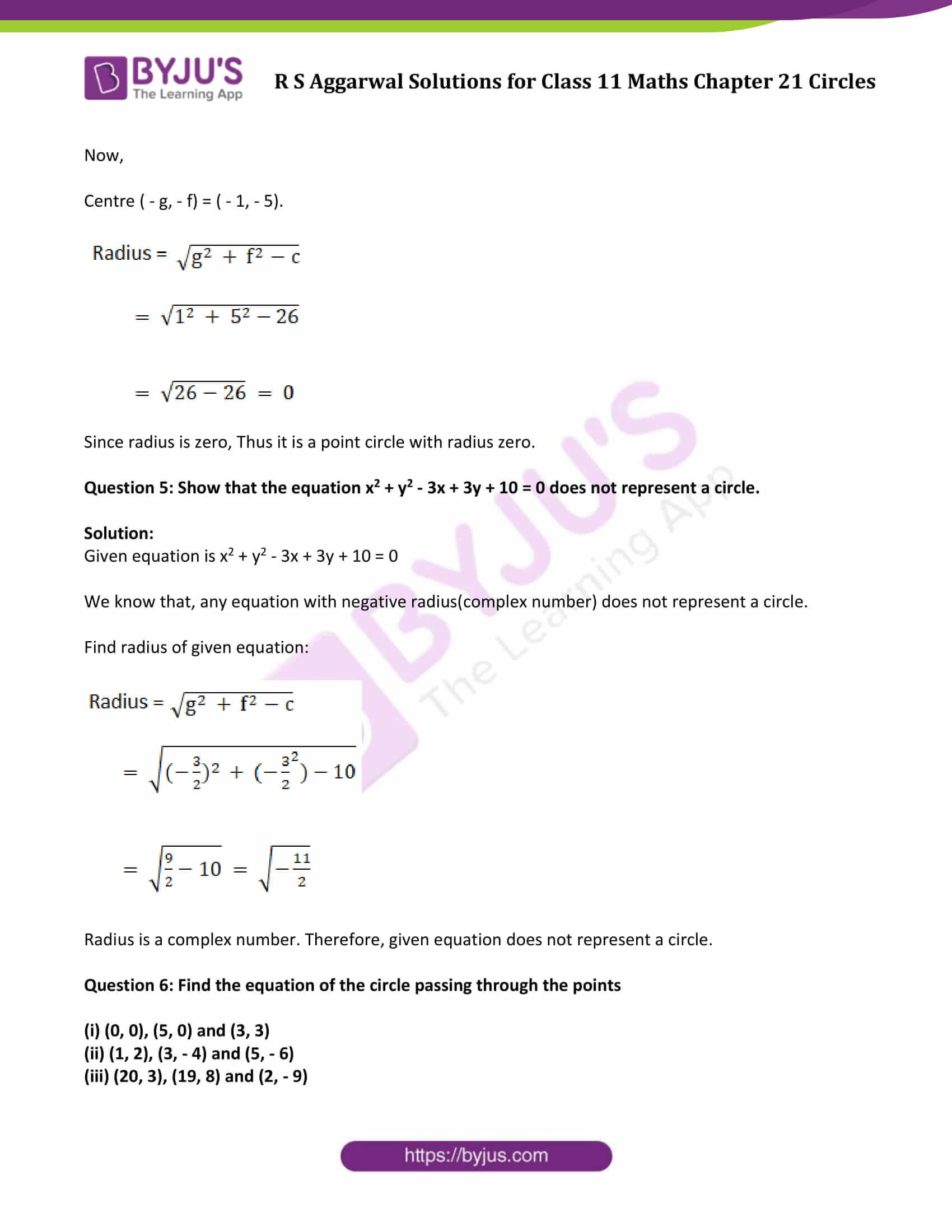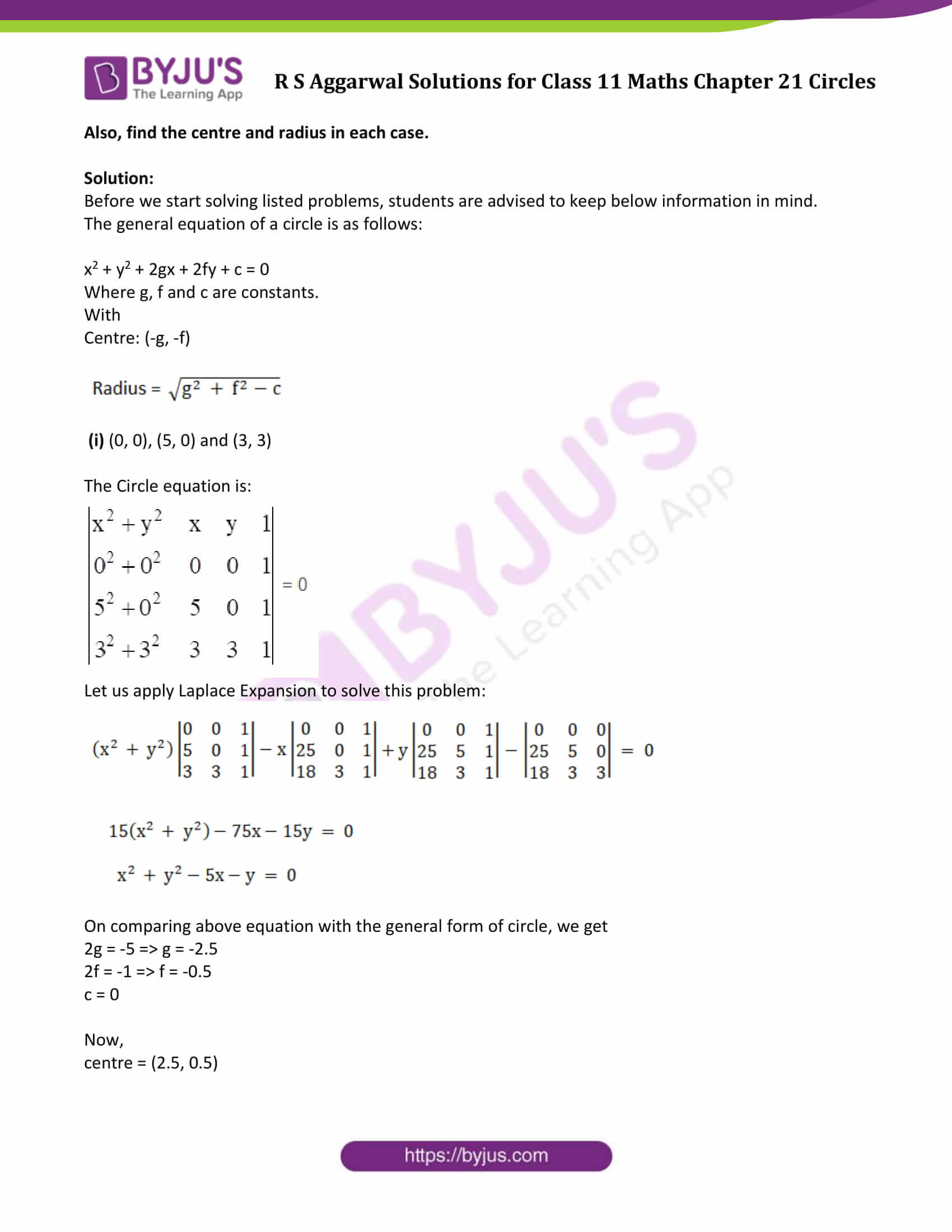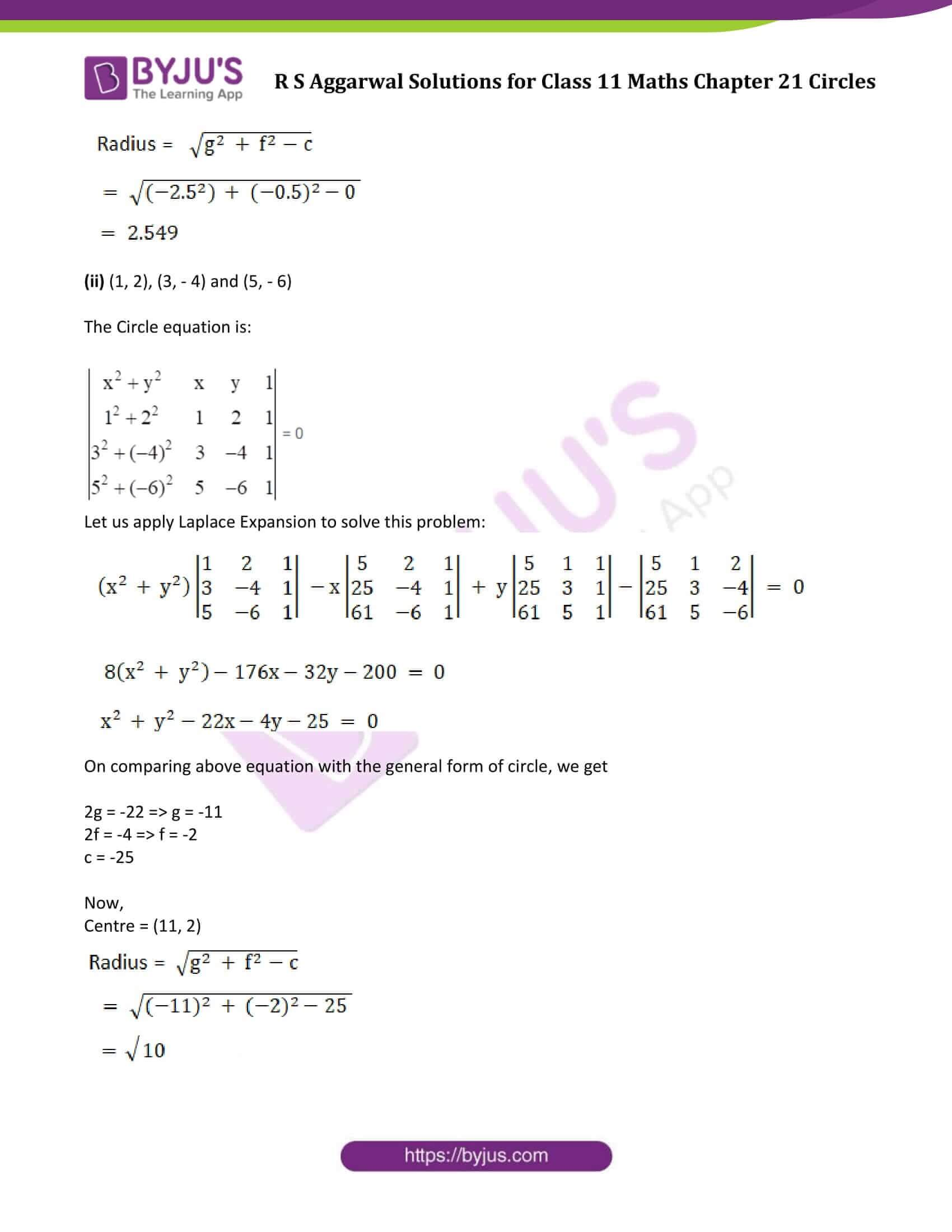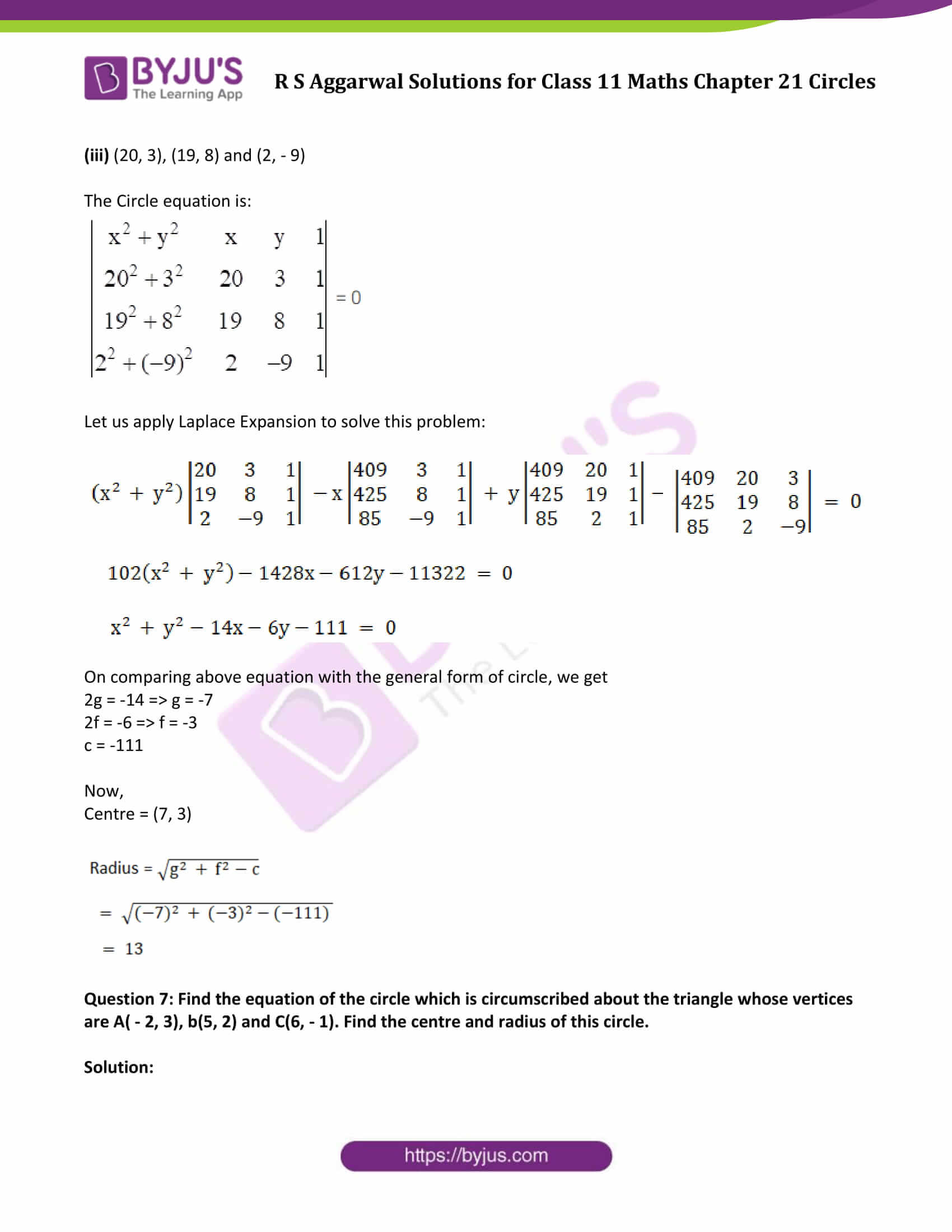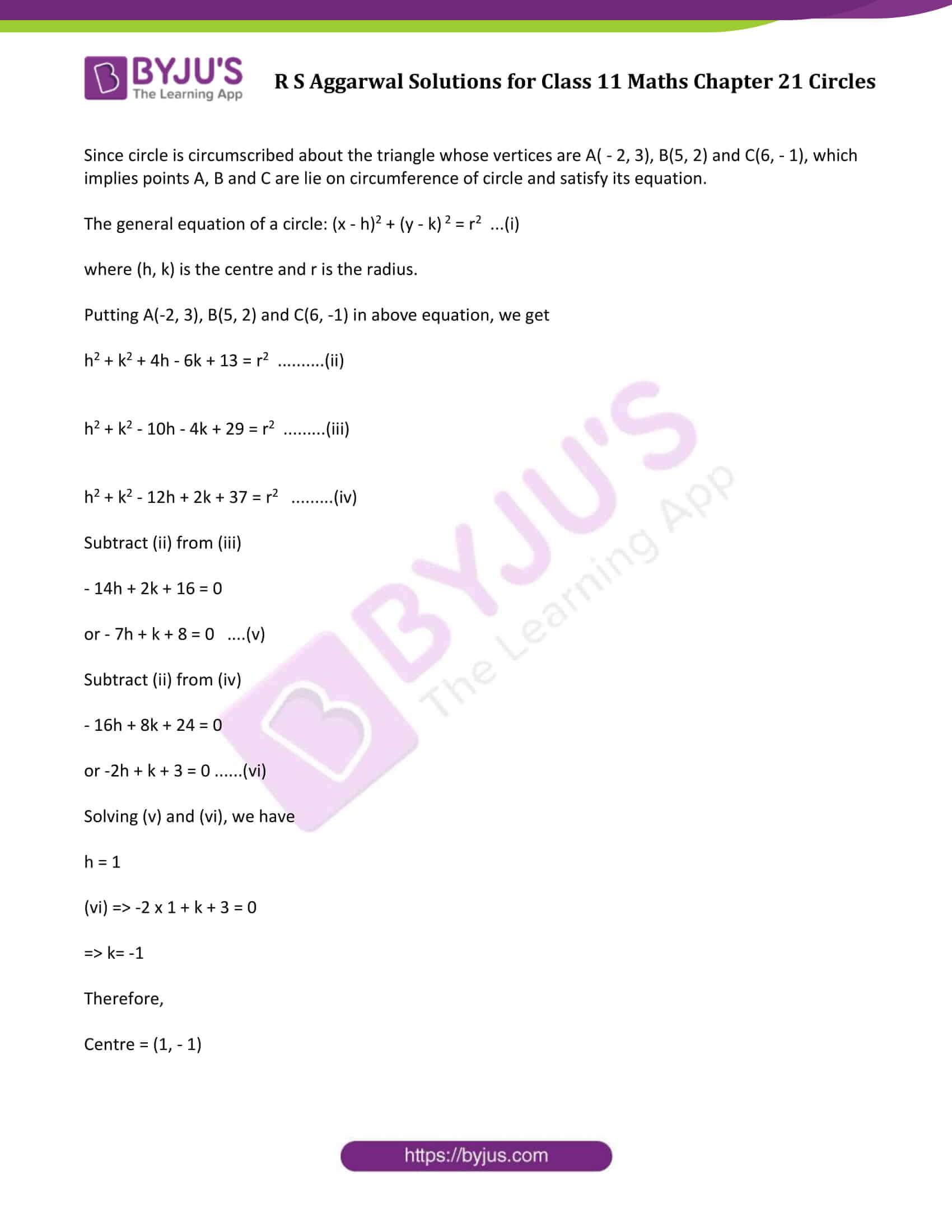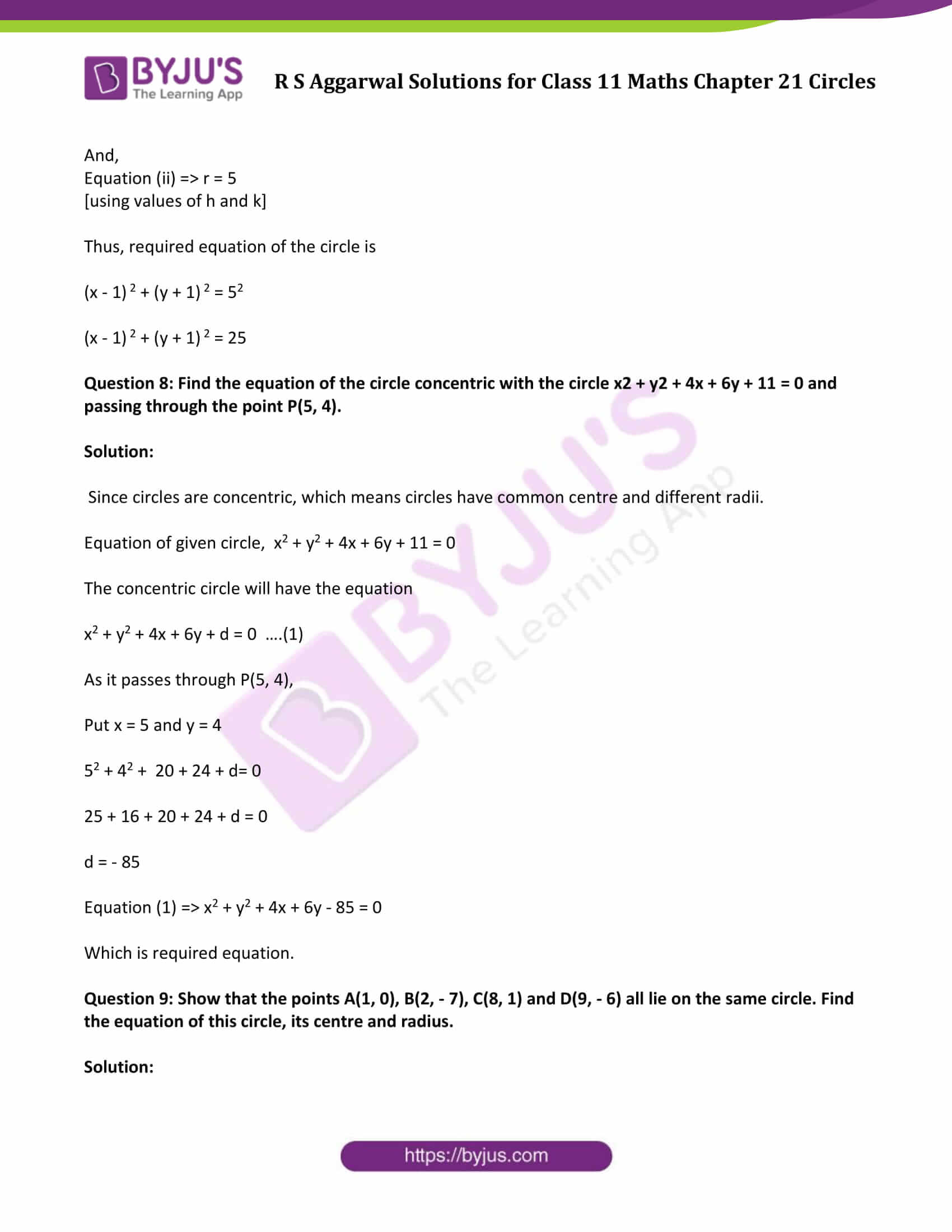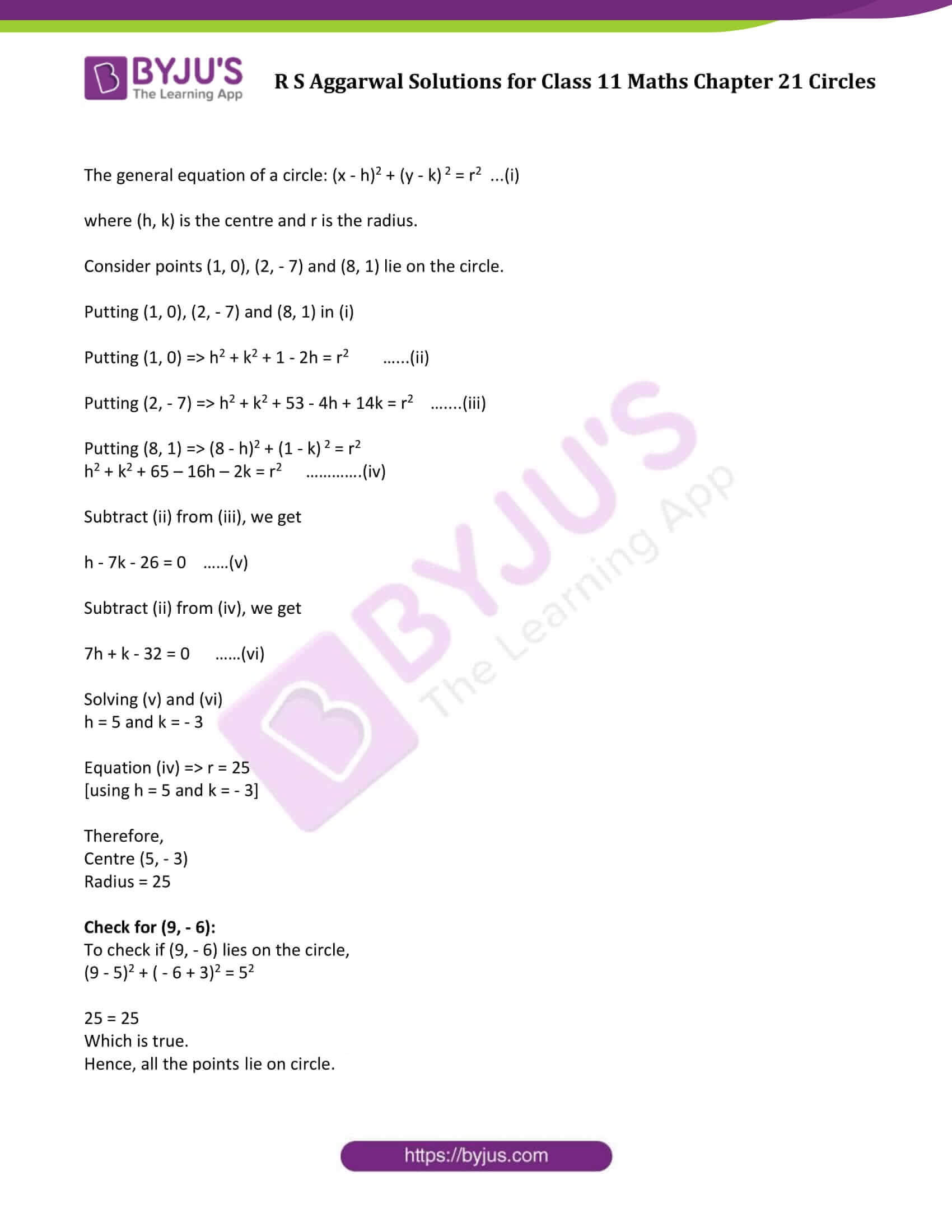### Access Answers of R S Aggarwal Solutions for Class 11 Maths Chapter 21 Circles Exercise 21B Page number 730

Exercise 21B Page No: 730

Question 1: Show that the equation x2 + y2 – 4x + 6y – 5 = 0 represents a circle. Find its centre and radius.

Solution:

Given equation is x2 + y2 – 4x + 6y – 5 = 0

The general equation of a circle is as follows:

x2 + y2 + 2gx + 2fy + c = 0

Where g, f and c are constants

With

Centre: (-g, -f)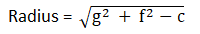On comparing given equation with general form of circle, we have

2g = – 4 ⇒ g = -2

2f = 6 ⇒ f = 3 and

c = – 5

Centre: (-g, -f) = (2, -3)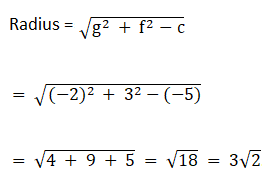Question 2: Show that the equation x2 + y2 + x – y = 0 represents a circle. Find its centre and radius.

Solution:

Given equation is x2 + y2 + x – y = 0

The general equation of a circle is as follows:

x2 + y2 + 2gx + 2fy + c = 0

Where g, f and c are constants

With

Centre: (-g, -f)On comparing given equation with general form of circle, we have

2g = 1 ⇒ g = 1/2

2f = – 1 ⇒ f = -1/2 and

c = 0

Now,

Centre: (-g, -f) = (-1/2, 1/2)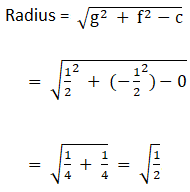Question 3: Show that the equation 3x2 + 3y2 + 6x – 4y – 1 = 0 represents a circle. Find its centre and radius.

Solution:

Given equation is 3x2 + 3y2 + 6x – 4y – 1 = 0

Or x2 + y2 + 2x – 4/3 y – 1/3 = 0

The general equation of a circle is as follows:

x2 + y2 + 2gx + 2fy + c = 0

Where g, f and c are constants.

With

Centre: (-g, -f)On comparing given equation with general form of circle, we have

2g = 2 ⇒ g = 1

2f = -4/3 ⇒ f = -2/3 and

c = -1/3

Now,

Centre (-g, -f) = (-1, 2/3)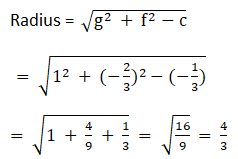Question 4: Show that the equation x2 + y2 + 2x + 10y + 26 = 0 represents a point circle. Also, find its centre.

Solution:

Given equation is x2 + y2 + 2x + 10y + 26 = 0

The general equation of a circle:

x2 + y2 + 2gx + 2fy + c = 0

where c, g, f are constants.

On comparing given equation with general equation of circle, we have

2g = 2 ⇒ g = 1

2f = 10 ⇒ f = 5 and

c = 26

Now,

Centre ( – g, – f) = ( – 1, – 5).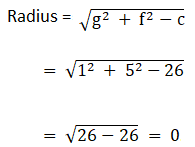Since radius is zero, Thus it is a point circle with radius zero.

Question 5: Show that the equation x2 + y2 – 3x + 3y + 10 = 0 does not represent a circle.

Solution:

Given equation is x2 + y2 – 3x + 3y + 10 = 0

We know that, any equation with negative radius(complex number) does not represent a circle.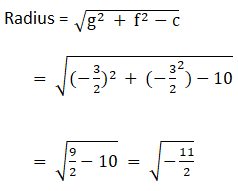Radius is a complex number. Therefore, given equation does not represent a circle.

Question 6: Find the equation of the circle passing through the points

(i) (0, 0), (5, 0) and (3, 3)

(ii) (1, 2), (3, – 4) and (5, – 6)

(iii) (20, 3), (19, 8) and (2, – 9)

Also, find the centre and radius in each case.

Solution:

Before we start solving listed problems, students are advised to keep below information in mind.

The general equation of a circle is as follows:

x2 + y2 + 2gx + 2fy + c = 0

Where g, f and c are constants.

With

Centre: (-g, -f)(i) (0, 0), (5, 0) and (3, 3)

The Circle equation is: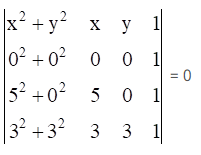Let us apply Laplace Expansion to solve this problem: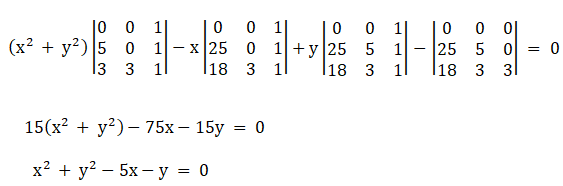On comparing above equation with the general form of circle, we get

2g = -5 ⇒ g = -2.5

2f = -1 ⇒ f = -0.5

c = 0

Now,

centre = (2.5, 0.5)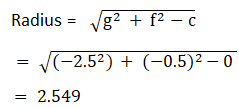(ii) (1, 2), (3, – 4) and (5, – 6)

The Circle equation is: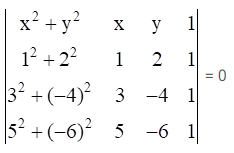Let us apply Laplace Expansion to solve this problem: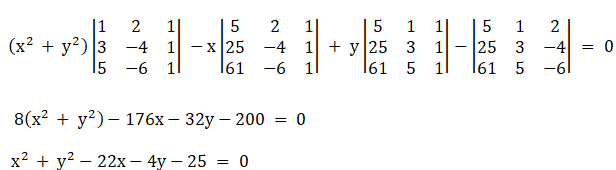On comparing above equation with the general form of circle, we get

2g = -22 ⇒ g = -11

2f = -4 ⇒ f = -2

c = -25

Now,

Centre = (11, 2)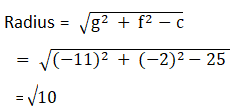(iii) (20, 3), (19, 8) and (2, – 9)

The Circle equation is: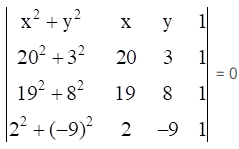Let us apply Laplace Expansion to solve this problem: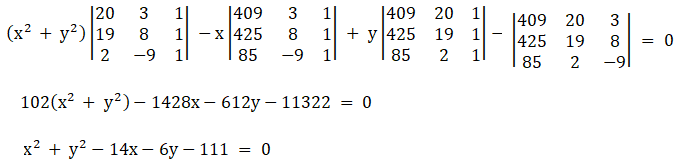On comparing above equation with the general form of circle, we get

2g = -14 ⇒ g = -7

2f = -6 ⇒ f = -3

c = -111

Now,

Centre = (7, 3)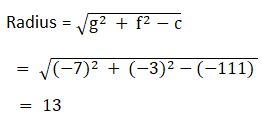Question 7: Find the equation of the circle which is circumscribed about the triangle whose vertices are A( – 2, 3), b(5, 2) and C(6, – 1). Find the centre and radius of this circle.

Solution:

Since circle is circumscribed about the triangle whose vertices are A( – 2, 3), B(5, 2) and C(6, – 1), which implies points A, B and C are lie on circumference of circle and satisfy its equation.

The general equation of a circle: (x – h)2 + (y – k) 2 = r2 …(i)

where (h, k) is the centre and r is the radius.

Putting A(-2, 3), B(5, 2) and C(6, -1) in above equation, we get

h2 + k2 + 4h – 6k + 13 = r2 ……….(ii)

h2 + k2 – 10h – 4k + 29 = r2 ………(iii)

h2 + k2 – 12h + 2k + 37 = r2 ………(iv)

Subtract (ii) from (iii)

– 14h + 2k + 16 = 0

or – 7h + k + 8 = 0 ….(v)

Subtract (ii) from (iv)

– 16h + 8k + 24 = 0

or -2h + k + 3 = 0 ……(vi)

Solving (v) and (vi), we have

h = 1

(vi) ⇒ -2 x 1 + k + 3 = 0

⇒ k= -1

Therefore,

Centre = (1, – 1)

And,

Equation (ii) ⇒ r = 5

[using values of h and k]

Thus, required equation of the circle is

(x – 1) 2 + (y + 1) 2 = 52

(x – 1) 2 + (y + 1) 2 = 25

Question 8: Find the equation of the circle concentric with the circle x2 + y2 + 4x + 6y + 11 = 0 and passing through the point P(5, 4).

Solution:

Since circles are concentric, which means circles have common centre and different radii.

Equation of given circle, x2 + y2 + 4x + 6y + 11 = 0

The concentric circle will have the equation

x2 + y2 + 4x + 6y + d = 0 ….(1)

As it passes through P(5, 4),

Put x = 5 and y = 4

52 + 42 + 20 + 24 + d= 0

25 + 16 + 20 + 24 + d = 0

d = – 85

Equation (1) ⇒ x2 + y2 + 4x + 6y – 85 = 0

Which is required equation.

Question 9: Show that the points A(1, 0), B(2, – 7), C(8, 1) and D(9, – 6) all lie on the same circle. Find the equation of this circle, its centre and radius.

Solution:

The general equation of a circle: (x – h)2 + (y – k) 2 = r2 …(i)

where (h, k) is the centre and r is the radius.

Consider points (1, 0), (2, – 7) and (8, 1) lie on the circle.

Putting (1, 0), (2, – 7) and (8, 1) in (i)

Putting (1, 0) ⇒ h2 + k2 + 1 – 2h = r2 ……(ii)

Putting (2, – 7) ⇒ h2 + k2 + 53 – 4h + 14k = r2 …….(iii)

Putting (8, 1) ⇒ (8 – h)2 + (1 – k) 2 = r2

h2 + k2 + 65 – 16h – 2k = r2 ………….(iv)

Subtract (ii) from (iii), we get

h – 7k – 26 = 0 ……(v)

Subtract (ii) from (iv), we get

7h + k – 32 = 0 ……(vi)

Solving (v) and (vi)

h = 5 and k = – 3

Equation (iv) ⇒ r = 25

[using h = 5 and k = – 3]

Therefore,

Centre (5, – 3)

Check for (9, – 6):

To check if (9, – 6) lies on the circle,

(9 – 5)2 + ( – 6 + 3)2 = 52

25 = 25

Which is true.

Hence, all the points lie on circle.

## Access other exercise solutions of Class 11 Maths Chapter 21 Circles

Exercise 21A Solutions

## R S Aggarwal Solutions for Class 11 Maths Chapter 21 Exercise 21B

Class 11 Maths Chapter 21 Circles Exercise 21B is based on general equation of a circle. Students can download R S Aggarwal chapter 21 solutions and practice more problems on circle.